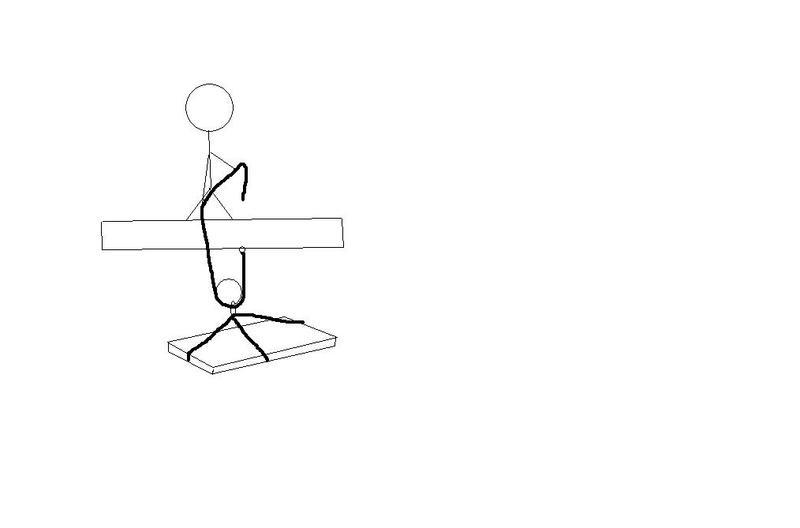# Finding the time in a tension problem

Hi I am new to this site. I have been stuck on this homework question for quite some time. A construction worker uses a rope and pulley system to hoist material. The rope is rated to withstand 500N of tension. What is the minimum time the construction worker can hoist 93 kg of material, intially at rest, 8 m up without exceeding the rated tension of the rope? neglect friction and the intertia of the rope and pulley

To be quite honest I don't even know where to start with this problem! Any help or advice would be greatly appreciated thank you

Doc Al
Mentor
Hint: What's the maximum allowable acceleration of the material?

wouldnt that be 9.81 m/s^2 but how can I incorporate this into the problem?

Doc Al
Mentor
wouldnt that be 9.81 m/s^2
No, you have to solve for the acceleration using Newton's 2nd law. What forces act on the material as it is being raised?

Once you find the maximum acceleration, use kinematics to solve for the time.

gravity-9.81 m/s^2(down)and the tension 500N (up)

Doc Al
Mentor
gravity-9.81 m/s^2(down)and the tension 500N (up)
Almost right. Gravity acts down with a force (not an acceleration) equal to the material's weight. (What's the weight of the material?)

93 kg

Doc Al
Mentor
93 kg
That's the mass. Use it to figure out the force of gravity (the weight).

93*9.81=912.33 n

Doc Al
Mentor
93*9.81=912.33 n
Looks good. Keep going.

okay from here Fnet=T-Fg 412.33=93kg(a) =412.33/93= 4.43 m/s^2

Doc Al
Mentor
The rope is rated to withstand 500N of tension.
Are you sure that the max tension is only 500 N? That's not even equal to the weight of the material. (Sure it's not 5000 N?)

Yes I am postive that part is straight out of the book, then the teachers typed question is "what is the minimum time the construction worker in prob 70(which states the 500N tension rating of the rope) can hoist 93 kg of material, intially at rest, 8 m up, without exceeding the rated tension of the rope. The final answer is 4.10 seconds but I obviously need to show how to get there

Doc Al
Mentor
Yes I am postive that part is straight out of the book,
Then we need more information, such as the exact arrangement of ropes and pulley. (Post a diagram.) You need to determine the force exerted on the material when the rope is at maximum tension.

Sorry I did not realize it was of great significanceThis is a construction worker ussing a rope and pulley system to hoist construction material to an upper floor

Doc Al
Mentor
Good. If the rope tension is 500 N, how much force do the ropes exert up on the pulley/material?

I am not quite sure would I divide the tension of 500N by the weight of 93 kg and get 5.4m/s^2 since would the force be 500 N on the object?

Last edited:
Doc Al
Mentor
I am not quite sure would I divide the tension of 500N by the weight of 93 kg and get 5.4m/s^2 since would the force be 500 N on the object?
No. You aren't ready to calculate the acceleration yet, you still have to determine the net force on the material.

Analyze the diagram and figure out how much upward force is exerted on the pulley. Hint: Count how many times the rope pulls up.